# Writing dataframes into an Excel template

People who are familiar with openpyxl know that we can use it to read/write Excel 2010 xlsx/xlsm/xltx/xltm files. In this blog, I will show you how to write values into an Excel template.

## Introduction

People who are familiar with openpyxl know that we can use it to read/write Excel 2010 xlsx/xlsm/xltx/xltm files. As I presented in this blog, we can create a workbook, assign values to some cells, apply number formats, merge cells, etc. However, if we need to create an Excel dashboard as the following, should we accomplish all formats with openpyxl?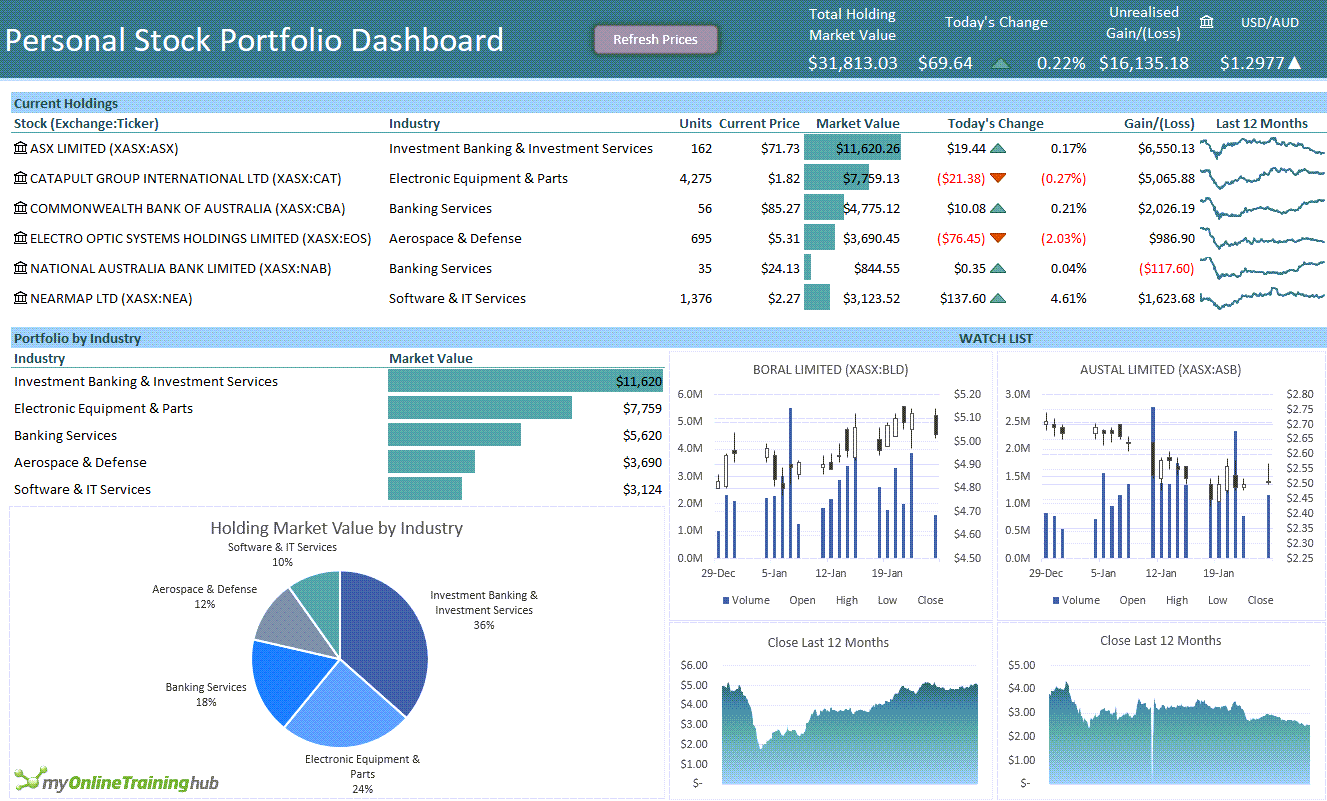For the question above, we can resolve it from another point of view: we can create an Excel template with the fixed format, such as dashboard title and logo, subtitles, then write values into this template. In this blog, I’ll show you how to do this with the following points:

• Context
• Write data into the template

## Context

We have an Excel template named “template.xlsx”, which contains two worksheets “category” and “product”: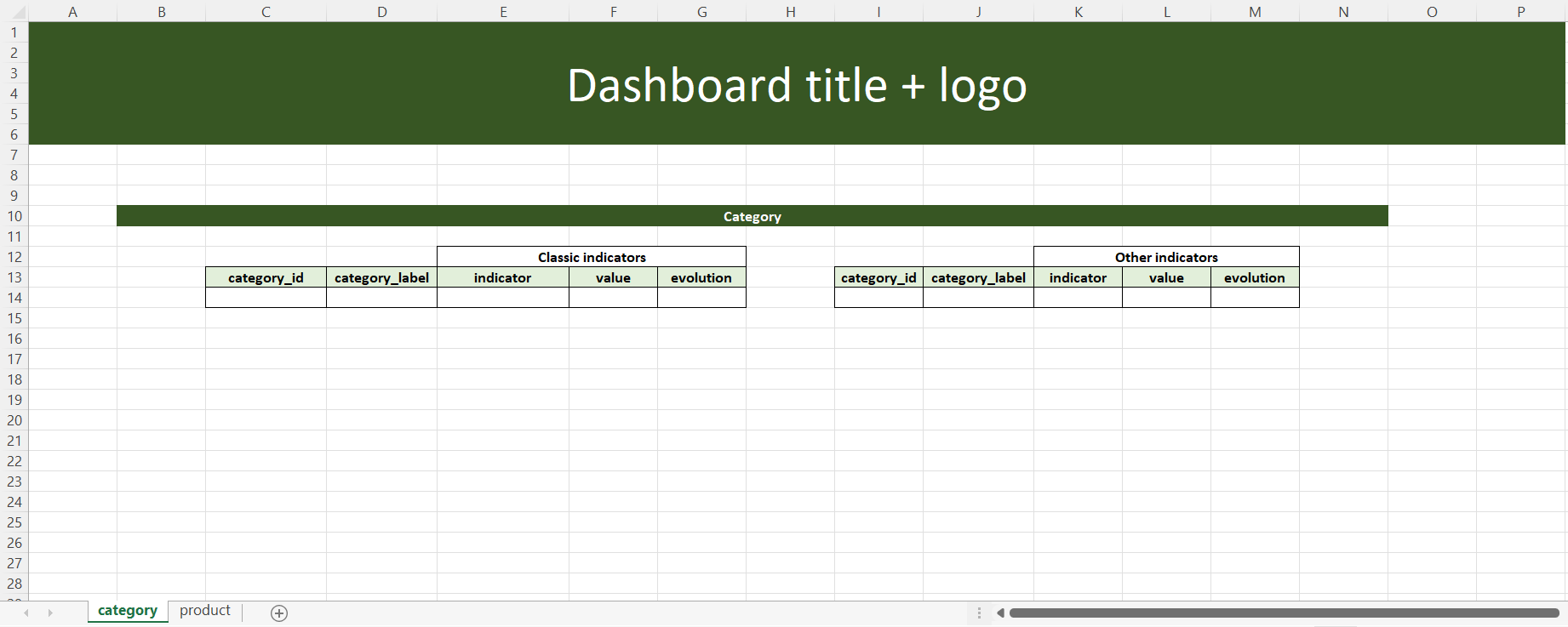The worksheet “category” shows the performance of each category with different indicators like turnover, volume, number of clients, etc.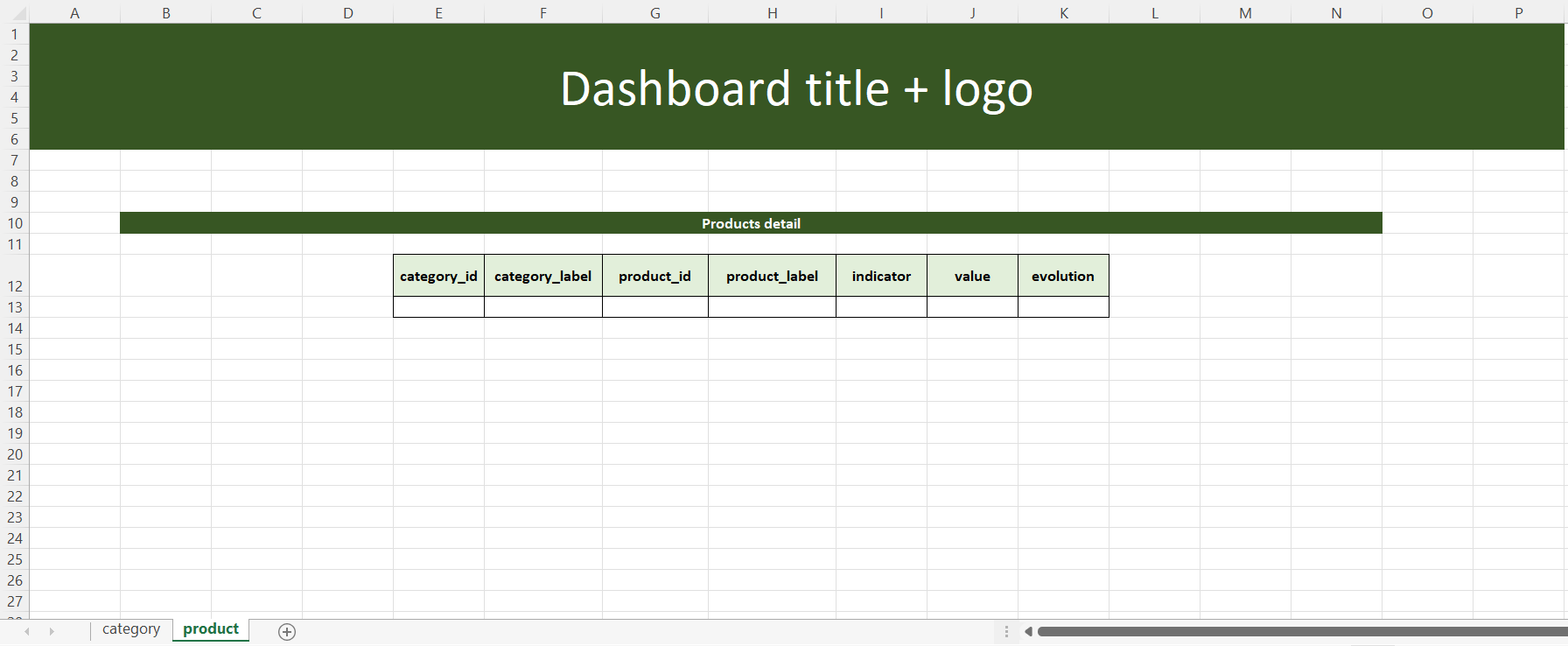The worksheet “product” shows the performance of each product with the same indicators.

And what we need to insert into these two worksheets are three pandas dataframes: classic_indicators_df, other_indicators_df and products_detail_df.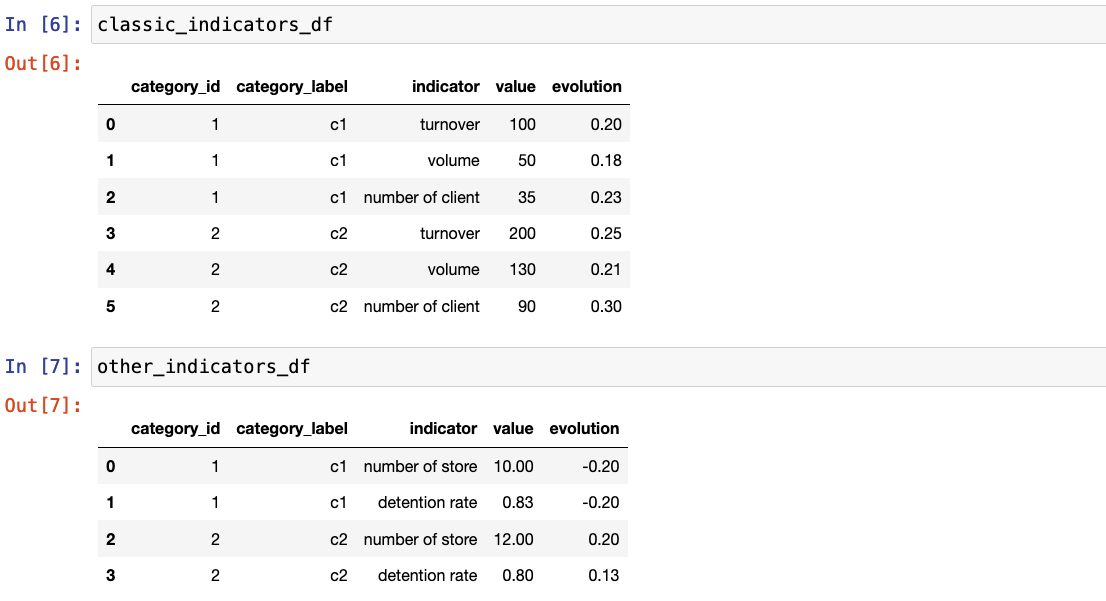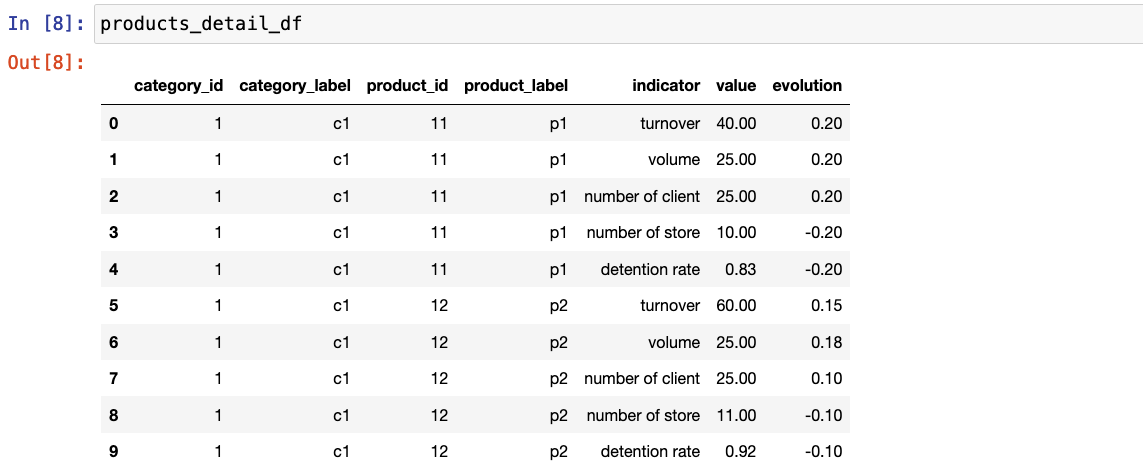## Write data into the template

With all data preparation, the next target is writing the three dataframes into the template with several steps:

• Create a writer
• Write dataframe into the template

template = openpyxl.load_workbook('./template.xlsx')


We load the template with openpyxl.load_workbook by indicating the path.

### Create a writer

import pandas as pd

out_path = './final_report.xlsx'

writer = pd.ExcelWriter(out_path)
writer.book = template


We set the writer with pandas.ExcelWriter that allows to write DataFrame objects into excel sheets, and set the template file as the writer’s workbook.

### Write dataframe into the template

import openpyxl
from openpyxl.styles.borders import Border, Side
import string

def set_border(ws, cell_range):
rows = ws[cell_range]
side = Side(border_style='thin', color="FF000000")

rows = list(rows)
max_y = len(rows) - 1  # index of the last row
for pos_y, cells in enumerate(rows):
max_x = len(cells) - 1  # index of the last cell
for pos_x, cell in enumerate(cells):
border = Border(
left=cell.border.left,
right=cell.border.right,
top=cell.border.top,
bottom=cell.border.bottom
)
border.left = side
border.right = side
border.top = side
border.bottom = side

cell.border = border


Before writing into the template, I create a function set_border() by using Side() and Border() to set borders for each cell in the given range.

df_sheet_list = [(classic_indicators_df, 'category'),
(other_indicators_df, 'category'),
(products_detail_df, 'product')]

for (df, sht) in df_sheet_list:
templ_sht = template[sht]
writer.sheets = {templ_sht.title:templ_sht}

if df is classic_indicators_df:
classic_indicators_df.to_excel(writer, sheet_name=sht, index=False,
set_border(writer.sheets[sht], f"C14:G{14-1+len(df)}")
elif df is other_indicators_df:
other_indicators_df.to_excel(writer, sheet_name=sht, index=False,

We assign the sheet where we will insert the dateframe to writer.sheets. Then we write the dataframe with .to_excel by specifying the writer we use, the worksheet that we want to insert, and the start row and the start column with integers, after all these steps, we save the file with writer.save().